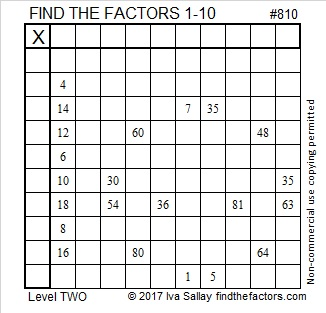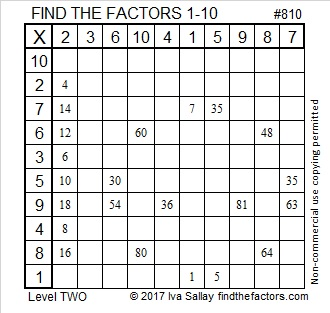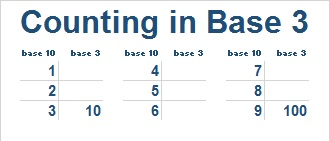# 810 Stick and StonePrint the puzzles or type the solution on this excel file: 10-factors 807-814One of my education professors taught that you can teach any concept with a picture book.

I recently read the book, Stick and Stone, to a class of 6th graders. Yes, 6th graders. You can get away with reading something way below grade level if you tell them before you start reading that you will use the book to introduce them to something that is definitely NOT below grade level. The first few pages of the book are shared by its publisher here:

As you can see, those first few pages equate stone as a zero and stick as a lonely number one.

The middle part of the book teaches about synergizing, working together to make life good and helping each other through tough times.

By the end of the book Stick and Stone know how to work very well together, “Stick, Stone. Together again. Stick, Stone. A perfect ten.”

The book pretty much ends there, but making a perfect ten is only the beginning of what these two characters can do together. I used this book to teach the class not only about getting along and working together, but also about base 2, or binary as it is also called. Every counting number we know can be represented by using just 1’s and 0’s. I wrote on the board the numbers from 1 to 16 and represented the first few of those numbers in base 2. Then I invited class members to come up with how to write the rest of the numbers in base 2. Some students caught on immediately while the others were able to learn how to do it by watching their classmates and listening to them. Eventually with at least 12 different student’s inputs, we came up with a chart that looked something like this:Notice that the numbers from 9 to 15 are just 1000 plus the numbers directly across from them in the first column.

Some of the sixth grade students had already heard of binary, so I showed them a little more about base 2: I wrote a bunch of 1’s and 0’s “off the top of my head” onto the board and added the headings to show place values: 1’s place, 2’s place, etc.Then I told them to sum up the place values that contained a one:Stick and Stone are the main two characters, but the book has one other character, Pinecone. At first Pinecone bullied Stone, but after Stick stood up to him, the three of them were eventually able to become friends. You might enjoy finding out more about Pinecone by listening to Sean Anderson read the entire book to his children, one of which seems to really enjoy numbers.

Children might also enjoy representing all the numbers from 1 to 31 with one hand:

If you used a unique symbol to represent Pinecone, it could look like a 2. Then you also could use the symbols 0, 1, and 2 to represent every counting number in base 3. That’s another concept the picture book Stick and Stone could be used to introduce!

To make a chart for base 3, start with these 3 columns of numbers with 3 numbers in each:Since this is base 3, where should 10 and 100 go? The bottom of the first column and the bottom of the third column both MUST look like a power of 10. The rest of the chart is easy to fill out. Notice the 1 and 2 look exactly the same in base 10 and base 3. Also since 4 = 3 + 1, 5 = 3 + 2, and 6 = 3 + 3, we can easily fill in the 2nd column. Two more addition facts will finish the third column: 7 = 6 + 1, and 8 = 6 + 2.Now add what you learned about 4, 5, 6, 7, 8, and 9 to column 1 and put the numbers 10 – 18 in the base 10 second column and numbers 19 – 27 in the base 10 third column. Again the bottom of the first column and the bottom of the third column both MUST look like a power of 10, so we now know where to put 1000.To fill in the rest of the chart simply add 100 to the base 3 numbers in column 1 to get the the base 3 numbers in column 2. Then add 200 to the base 3 numbers in column 1 to get the remaining base 3 numbers in column 3.You could do this process again to determine the first 81 counting numbers in base 3 with 81 being represented by 10000.

For base 4, you could do something similar with 4 columns. However, for counting in bases 4, 5, 6, 7, 8, and 9 I would suggest using the very versatile hundred chart. You can give instructions without even mentioning the concept of differing bases. For example, cross out every number on the hundred chart that has 7, 8, or 9 as one or more of its digits. Can you tell even before you get started how many numbers will get crossed out? (100 – 7²) What pattern do the cross-outs make? If you arrange the remaining numbers in order from smallest to largest, then you will have the first 49 numbers represented in base 7. With a minimal amount of cutting and taping you could have a “hundred” chart in base 7. Easy peasy.

This excel file not only has several puzzles, including today’s, but also a hundred chart and even a thousand chart because I know some of you might want to play with 3-digit numbers, too.

Now let me tell you a little bit about the number 810:

• 810 is a composite number.
• Prime factorization: 810 = 2 x 3 x 3 x 3 x 3 x 5, which can be written 810 = 2 × 3⁴ × 5
• The exponents in the prime factorization are 1, 4 and 1. Adding one to each and multiplying we get (1 + 1)(4 + 1)(1 + 1) = 2 x 5 x 2 = 20. Therefore 810 has exactly 20 factors.
• Factors of 810: 1, 2, 3, 5, 6, 9, 10, 15, 18, 27, 30, 45, 54, 81, 90, 135, 162, 270, 405, 810
• Factor pairs: 810 = 1 x 810, 2 x 405, 3 x 270, 5 x 162, 6 x 135, 9 x 90, 10 x 81, 15 x 54, 18 x 45 or 27 x 30
• Taking the factor pair with the largest square number factor, we get √810 = (√81)(√10) = 9√10 ≈ 28.4604989.Since 810 has so many factors, it has MANY possible factor trees. If most people made a factor tree for 810, they would probably start with 81 × 10 or 9 x 90. NOT ME! Here are two less-often-used factor trees for 810:Finally, here is an easy way to express 810 is in a different base:

1. Make a cake in which you divide 810 by the base number repeatedly, keeping track of the remainders, including zero, as you go.
2. Keep dividing until the number at the top of the cake is 0.
3. List the remainders in order from top to bottom and indicate the base you used to do the division.
4. This method is illustrated for BASE 2 and BASE 3 below:That’s all pretty good work for a stone, a stick, and a pine cone!

By the way, using that method will also produce the following results:

• 810 is 30222 BASE 4
• 810 is 11220 BASE 5
• 810 is 3430 BASE 6 and so forth.

And just so you’ll know, 810 is the sum of consecutive primes 401 and 409.

## One thought on “810 Stick and Stone”

1.The Chaos Realm

This site uses Akismet to reduce spam. Learn how your comment data is processed.